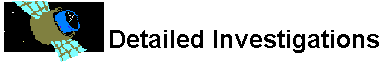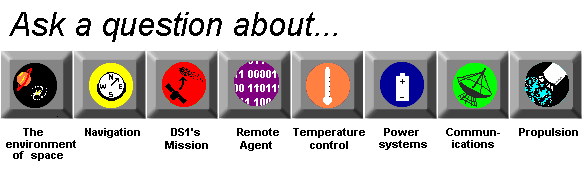# Propulsion

## How do you calculate rocket engine performance?

Rocket engine performance can be roughly calculated by the thrust equation:

T = mVe + (pe - pa) Ae
where
T = thrust force
m = flow rate = feVeAe
fe = fluid density at nozzle exit
Ve = exhaust velocity at nozzle exit
pe = exhaust pressure at nozzle exit
pa = ambient pressure
Ae = nozzle exit area

This means that the produced by a rocket is sum of two forces:

1. the flow rate * exhaust velocity
2. pressure difference * area of nozzle

However the dominant term (the one that is numerically much bigger than the other) is mVe, and therefore the thrust can sometimes be approximated as:

T = mVe

You can see that the major predictor of the performance of a rocket is how much stuff can you throw out the back multiplied by how fast you can throw it out. The larger the thrust force, the more mass the rocket can lift off the ground. However there are other factors that can affect the rocket engine performance.

 Rocket type Limiting factor Effects chemical rocket mass limited run out of stuff to throw out the back ion/electrical rocket energy limited not enough energy to throw out large amounts of propellant

The fundamental measure of rocket engine performance is how much mass can you propel out the back and at what speeds the propellant is driven out the back. Chemical rockets can spew a large quantity of propellant out at any one time, but because of this, they are less efficient then ion/electrical rockets which spew propellant out at higher speeds.How are rockets designed?How do conventional rockets work?What is thrust?How does a multi-stage rocket like a Delta II work?How fast do conventional rockets go?How fast does DS1 go?What is specific impulse?How do you calculate specific impulse?What are some rocket propellants?How does density affect tank volume?